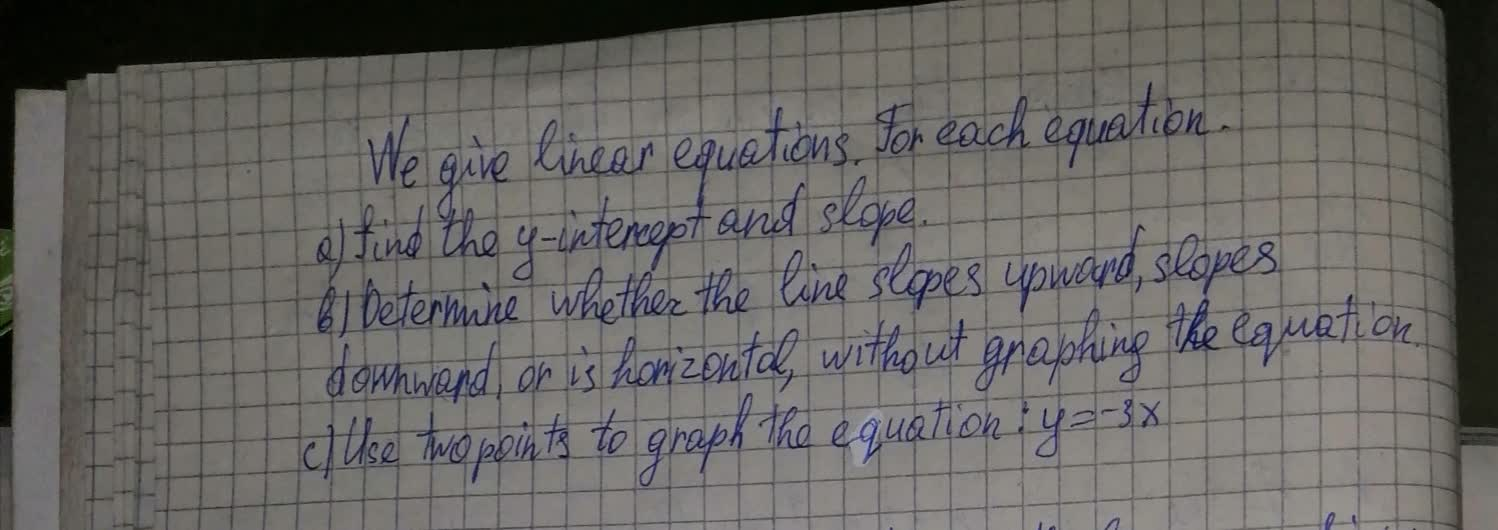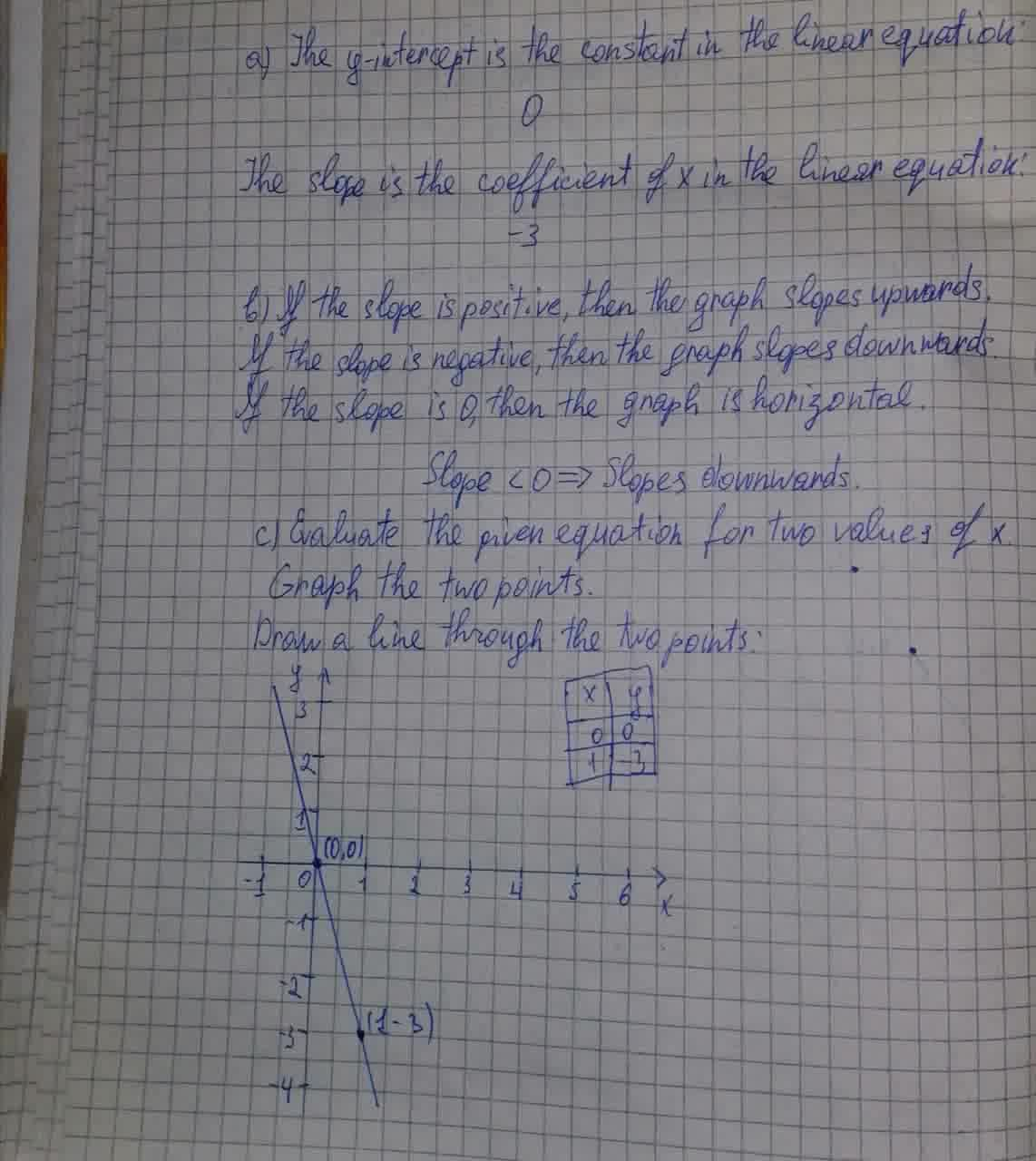Question# We give linear equation y=-3x

Second order linear equations
ANSWEREDWe give linear equations y=-3x